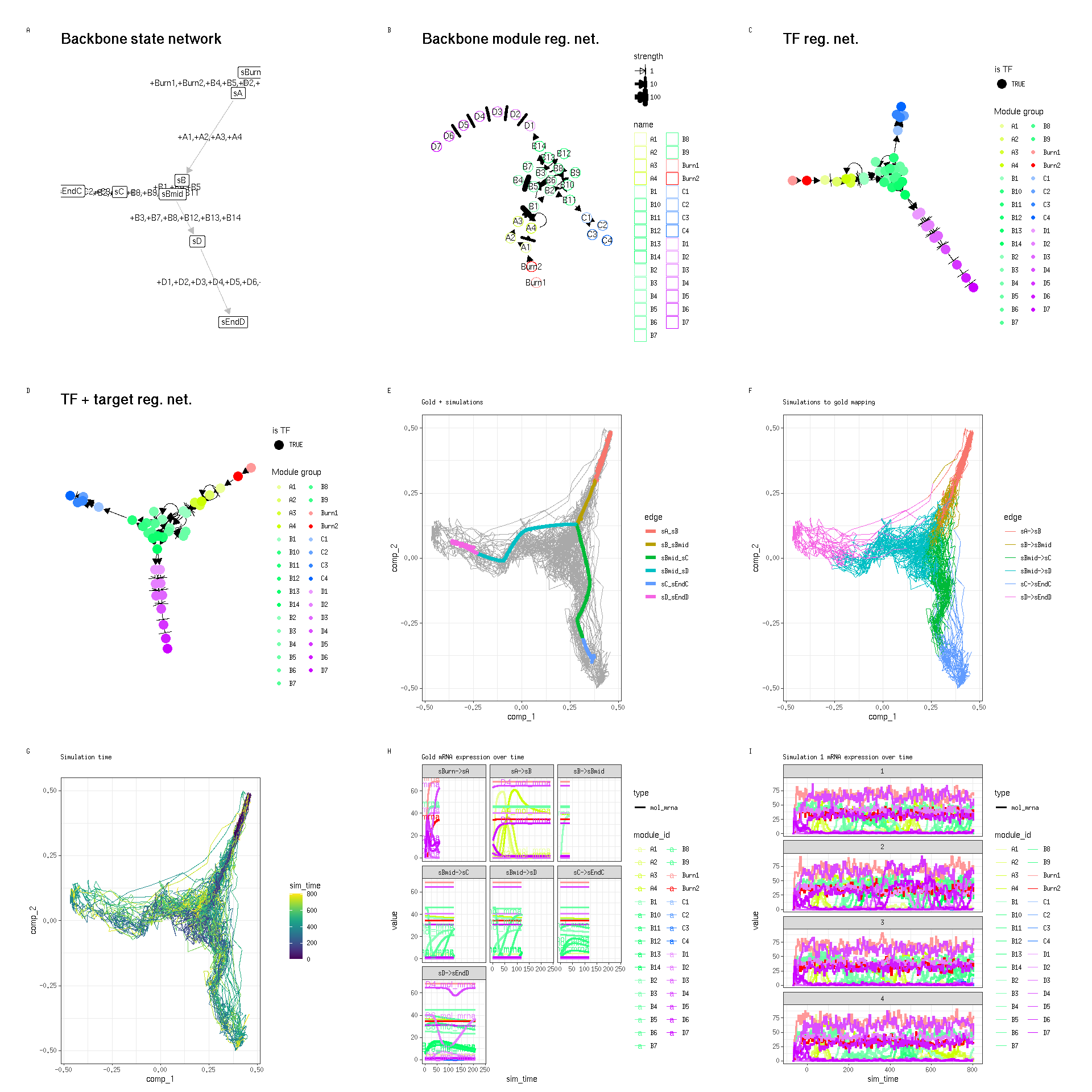# Step-by-step example run

## Step 1: Define backbone and other parameters

A dyngen simulation can be started by providing a backbone to the `initialise_model()` function. The backbone of a `dyngen` model is what determines the overall dynamic process that a cell will undergo during a simulation. It consists of a set of gene modules, which regulate eachother in such a way that expression of certain genes change over time in a specific manner.

``````library(tidyverse)
library(dyngen)

set.seed(10)
model <-
initialise_model(
num_tfs = 12,
num_targets = 30,
num_hks = 15,
backbone = backbone_bifurcating(),
verbose = TRUE,
num_cores = 8
)

plot_backbone_statenet(model)``````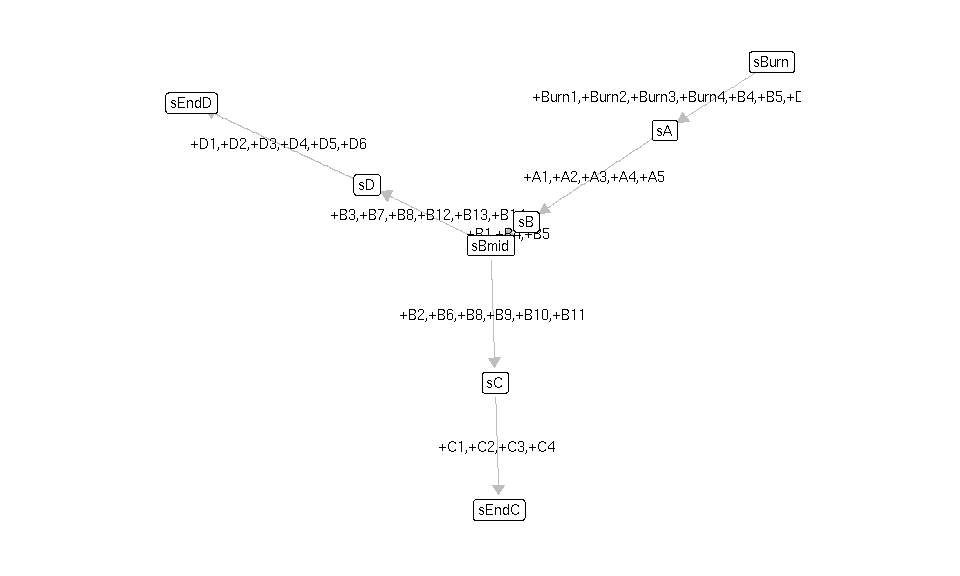``plot_backbone_modulenet(model)``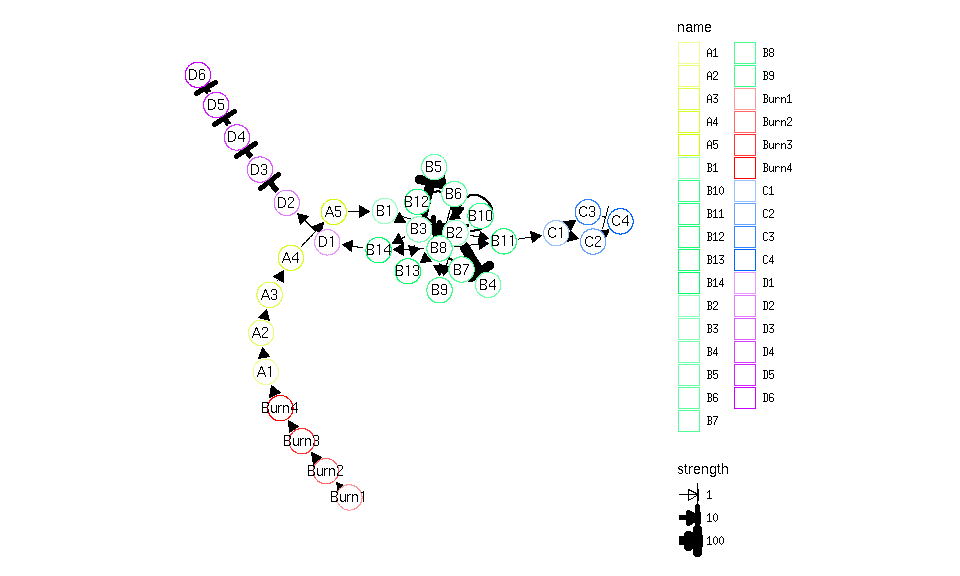For backbones with all different sorts of topologies, check `list_backbones()`:

``names(list_backbones())``
``````##   "bifurcating"             "bifurcating_converging"  "bifurcating_cycle"       "bifurcating_loop"        "binary_tree"
##   "branching"               "consecutive_bifurcating" "converging"              "cycle"                   "cycle_simple"
##  "disconnected"            "linear"                  "linear_simple"           "trifurcating"
``````

## Step 2: Generate transcription factors (TFs)

Each gene module consists of a set of transcription factors. These can be generated and visualised as follows.

``model <- generate_tf_network(model)``
``````## Generating TF network
``````
``plot_feature_network(model, show_targets = FALSE)``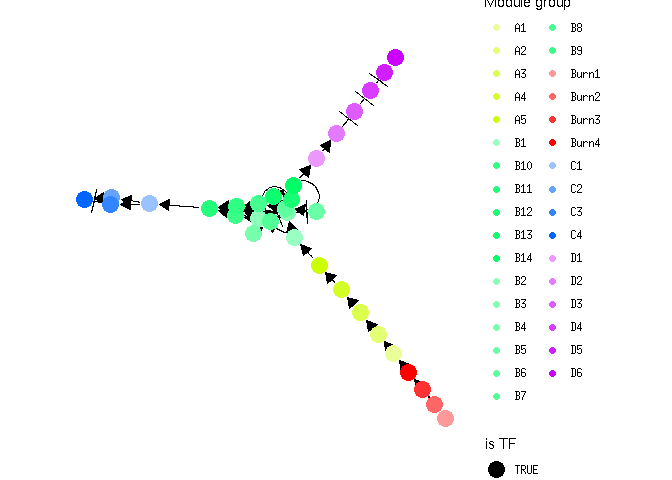## Step 3: Sample target genes and housekeeping genes (HKs)

Next, target genes and housekeeping genes are added to the network by sampling a gold standard gene regulatory network using the Page Rank algorithm. Target genes are regulated by TFs or other target genes, while HKs are only regulated by themselves.

``model <- generate_feature_network(model)``
``````## Sampling feature network from real network
``````
``plot_feature_network(model)``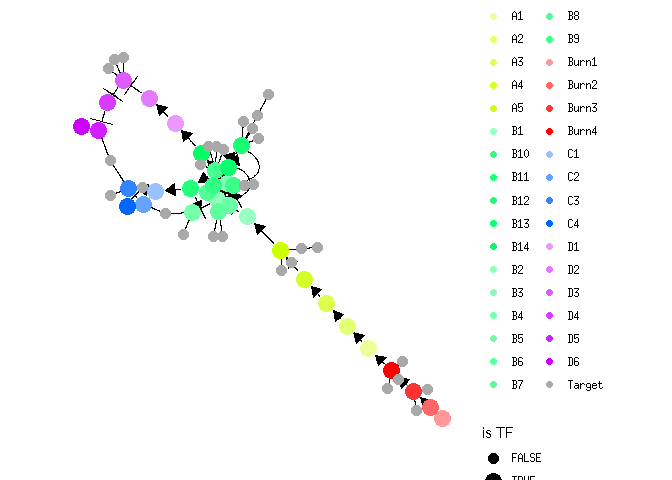``plot_feature_network(model, show_hks = TRUE)``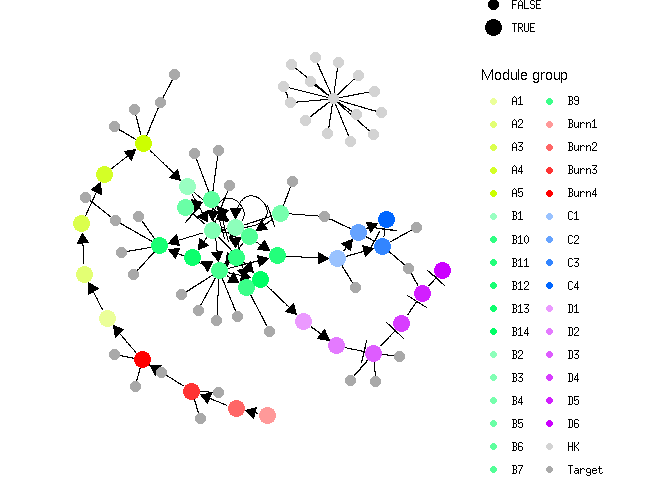## Step 4: Generate kinetics

Note that the target network does not show the effect of some interactions, because these are generated along with other kinetics parameters of the SSA simulation.

``model <- generate_kinetics(model)``
``````## Generating kinetics for 78 features
## Generating formulae
``````
``plot_feature_network(model)``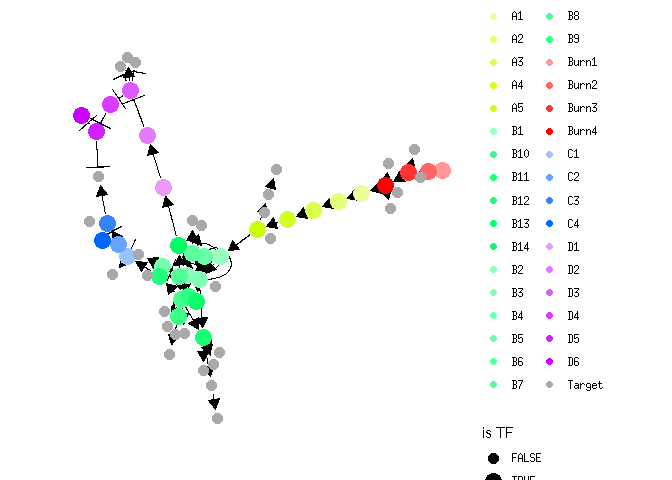``plot_feature_network(model, show_hks = TRUE)``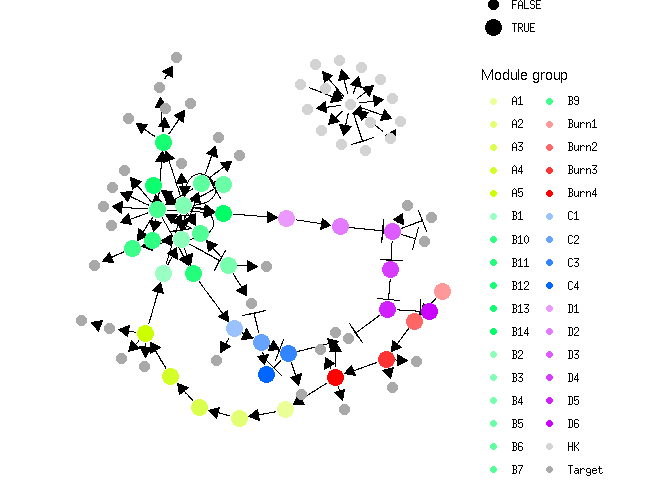## Step 5: Simulate gold standard

The gold standard is simulated by enabling certain parts of the module network and performing ODE simulations. The gold standard are visualised by performing a dimensionality reduction on the mRNA expression values.

``model <- generate_gold_standard(model)``
``````## Generating gold standard mod changes
## Precompiling reactions for gold standard
## Running gold simulations
##   |                                                  | 0 % elapsed=00s     |========                                          | 14% elapsed=00s, remaining~01s  |===============                                   | 29% elapsed=00s, remaining~01s  |======================                            | 43% elapsed=00s, remaining~00s  |=============================                     | 57% elapsed=00s, remaining~00s  |====================================              | 71% elapsed=01s, remaining~00s  |===========================================       | 86% elapsed=01s, remaining~00s  |==================================================| 100% elapsed=01s, remaining~00s
``````
``plot_gold_simulations(model) + scale_colour_brewer(palette = "Dark2")``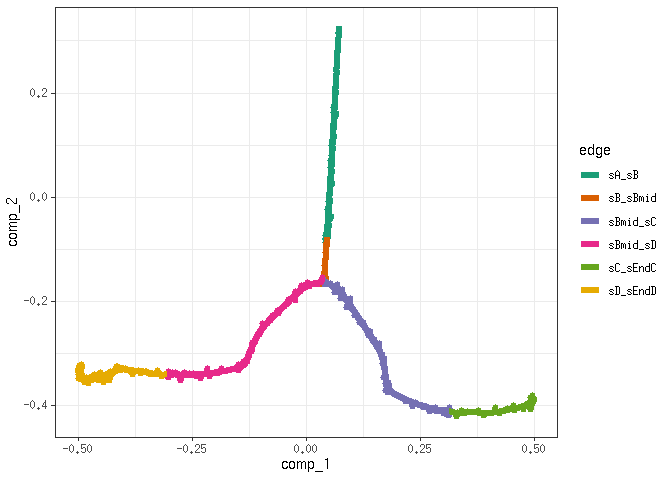The expression of the modules (average of TFs) can be visualised as follows.

``plot_gold_expression(model, what = "mol_mrna") # mrna``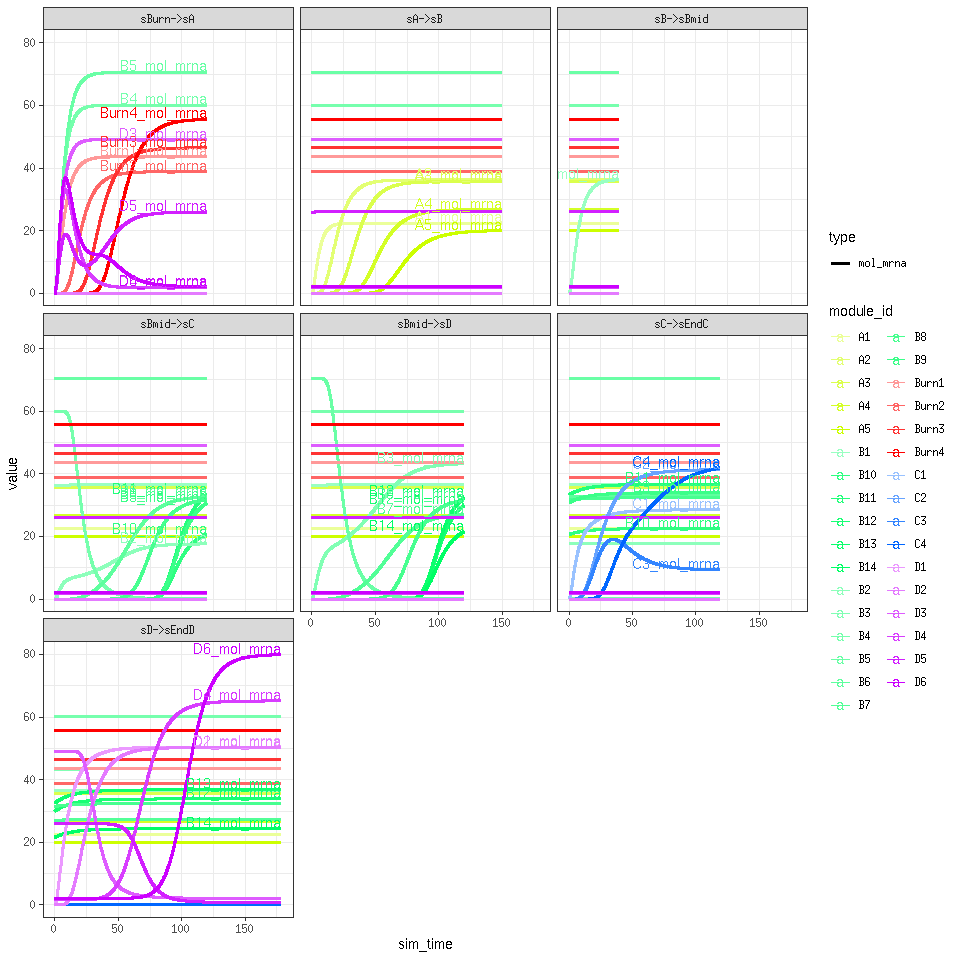``plot_gold_expression(model, label_changing = FALSE) # premrna, mrna, and protein``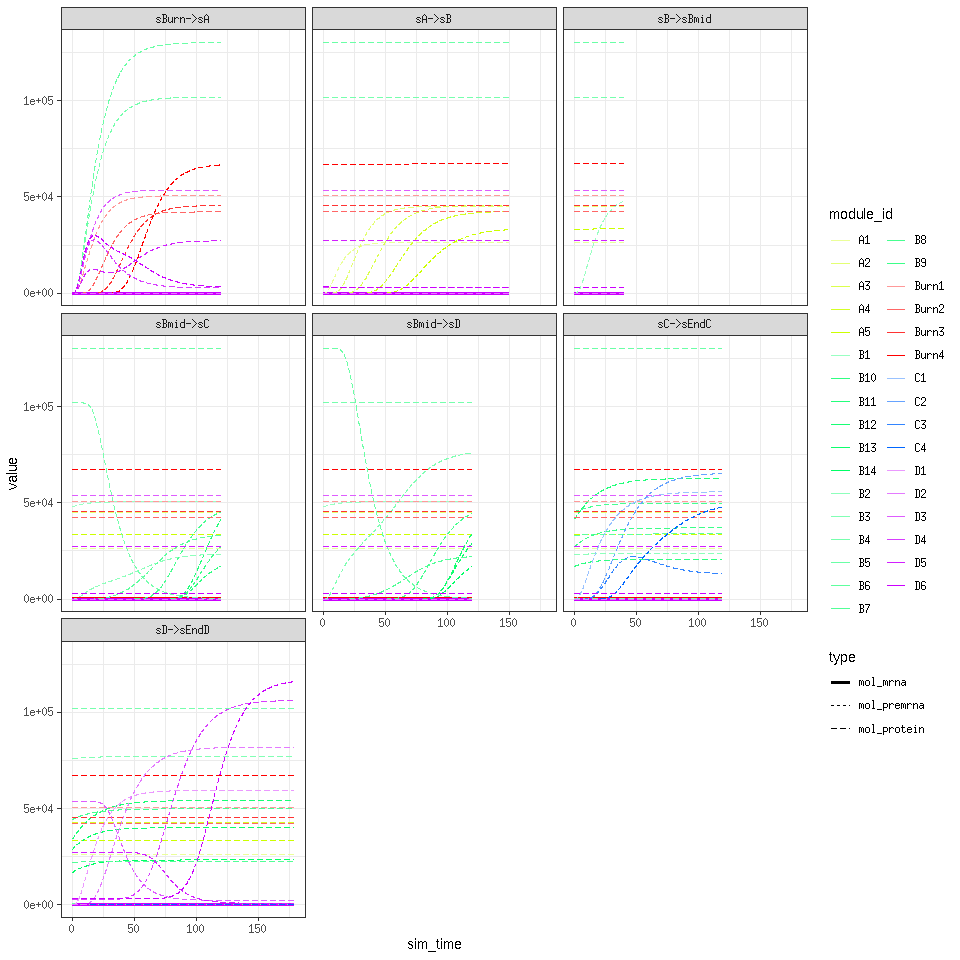## Step 6: Simulate cells.

Cells are simulated by running SSA simulations. The simulations are again using dimensionality reduction.

``model <- generate_cells(model)``
``````## Precompiling reactions for simulations
## Running 32 simulations
## Mapping simulations to gold standard
## Performing dimred
``````
``plot_simulations(model)``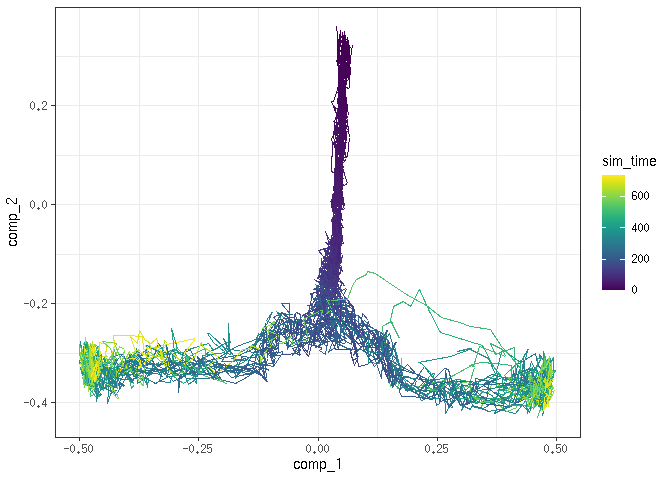The gold standard can be overlayed on top of the simulations.

``plot_gold_simulations(model) + scale_colour_brewer(palette = "Dark2")``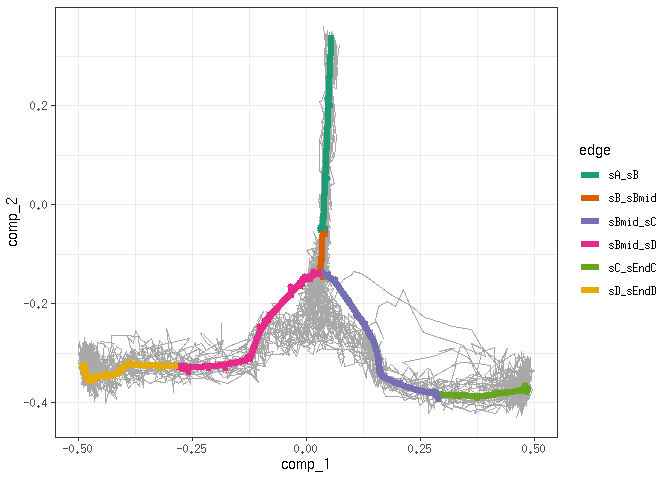We can check how each segment of a simulation is mapped to the gold standard.

``plot_gold_mappings(model, do_facet = FALSE) + scale_colour_brewer(palette = "Dark2")``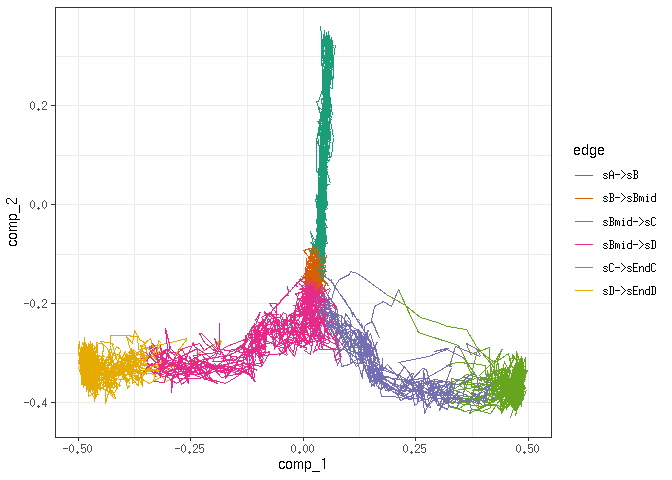The expression of the modules (average of TFs) of a single simulation can be visualised as follows.

``plot_simulation_expression(model, 1:4, what = "mol_mrna")``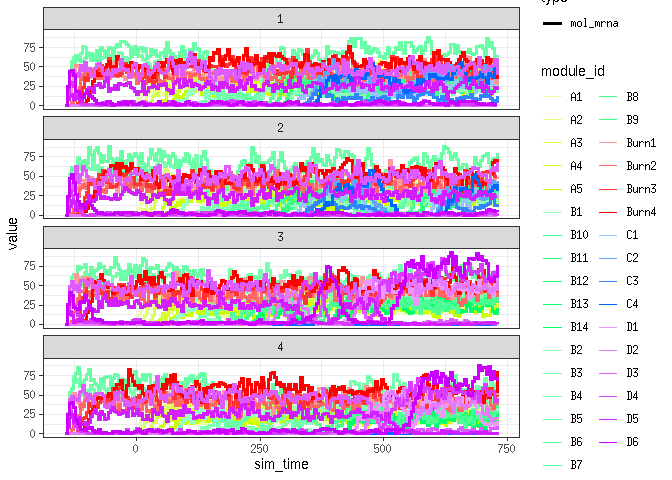## Step 7: Experiment emulation

Effects from performing a single-cell RNA-seq experiment can be emulated as follows.

``model <- generate_experiment(model)``
``````## Simulating experiment
``````

## Step 8: Convert to a dynwrap object

``dataset <- wrap_dataset(model)``

## Visualise with `dynplot`

``````library(dynplot)
plot_dimred(dataset)``````
``````## Coloring by milestone

## Using milestone_percentages from trajectory
``````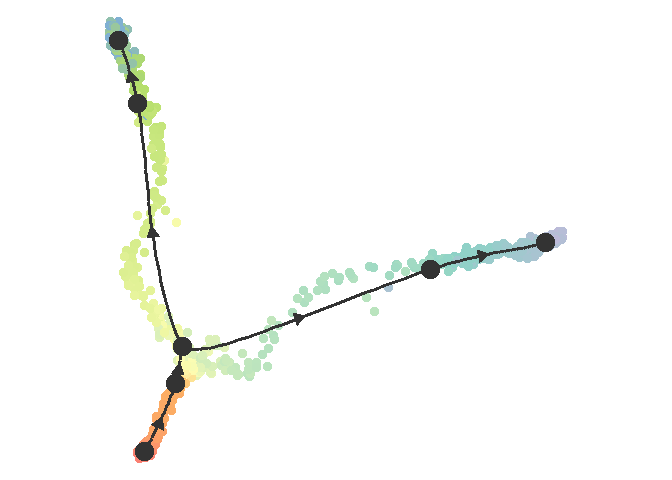``plot_graph(dataset)``
``````## Coloring by milestone
## Using milestone_percentages from trajectory
``````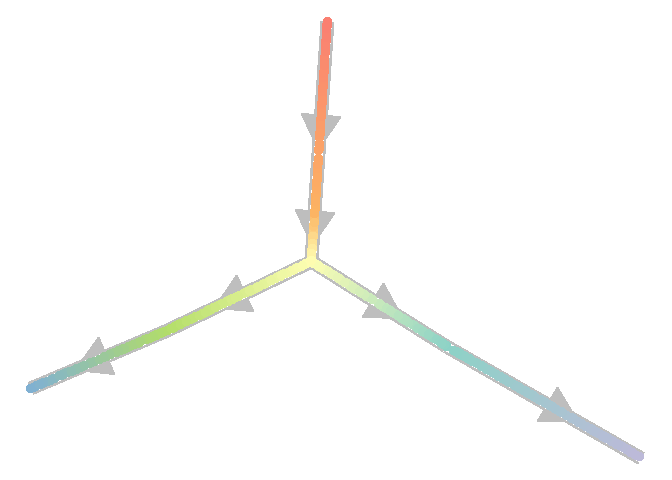## Infer trajectory on expression data

``````library(dyno)
pred <- infer_trajectory(dataset, ti_slingshot())``````
``````## Using full covariance matrix

## Warning in if (class(X) == "dist") X <- as.matrix(X): the condition has length > 1 and only the first element will be used
``````
``plot_dimred(pred)``
``````## Coloring by milestone

## Using milestone_percentages from trajectory
``````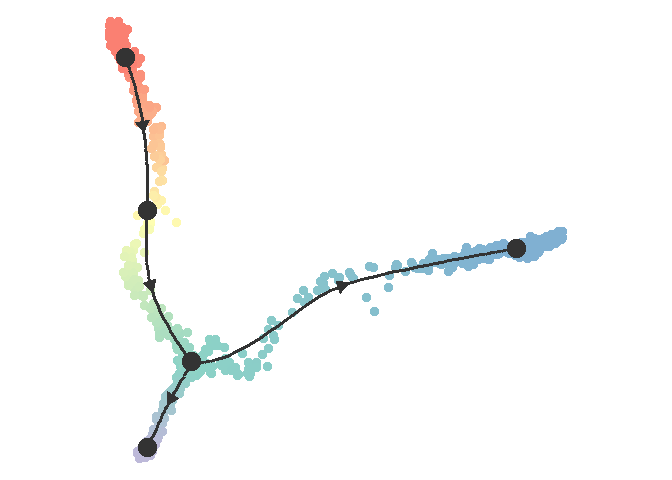# One-shot function

`dyngen` also provides a one-shot function for running all of the steps all at once and producing plots.

``````set.seed(1)
config <-
initialise_model(
num_tfs = 12,
num_targets = 30,
num_hks = 15,
backbone = backbone_bifurcating_converging(),
verbose = FALSE,
num_cores = 8
)

out <- generate_dataset(
config,
make_plots = TRUE
)``````
``````dataset <- out\$dataset
model <- out\$model
print(out\$plot)``````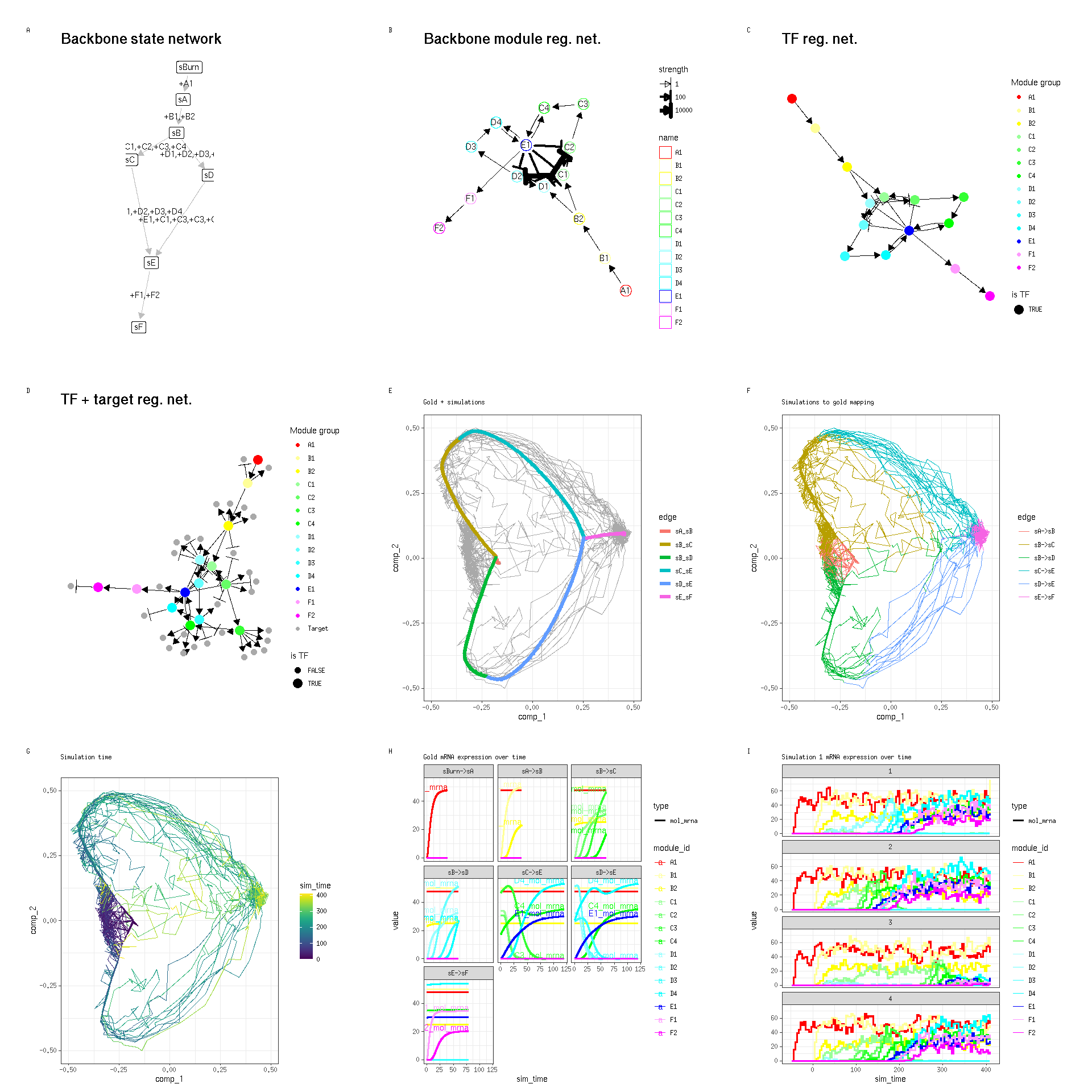`dataset` and `model` can be used in much the same way as before.

``plot_dimred(dataset)``
``````## Coloring by milestone

## Using milestone_percentages from trajectory
``````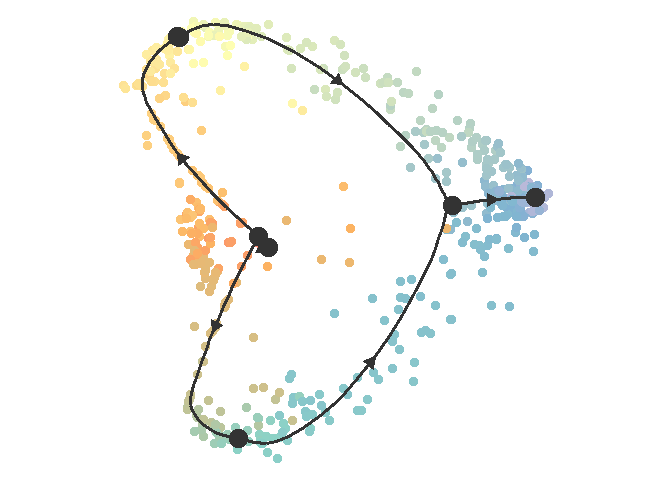``plot_graph(dataset)``
``````## Coloring by milestone
## Using milestone_percentages from trajectory
``````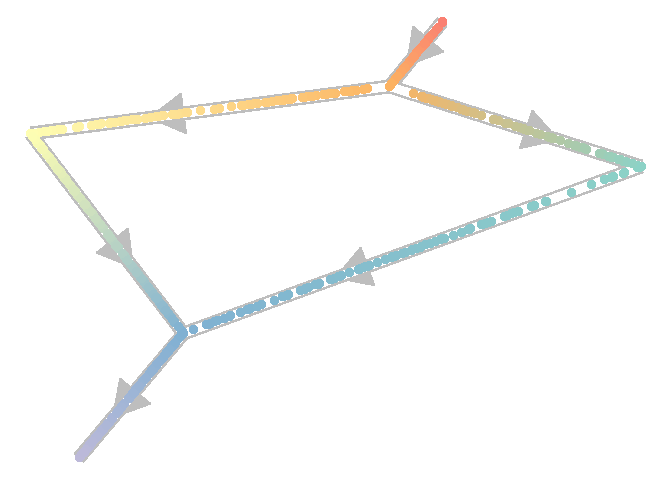``pred <- infer_trajectory(dataset, ti_slingshot(), verbose = FALSE)``
``````## Using full covariance matrix

## Warning in if (class(X) == "dist") X <- as.matrix(X): the condition has length > 1 and only the first element will be used
``````
``plot_dimred(pred)``
``````## Coloring by milestone
## Using milestone_percentages from trajectory
``````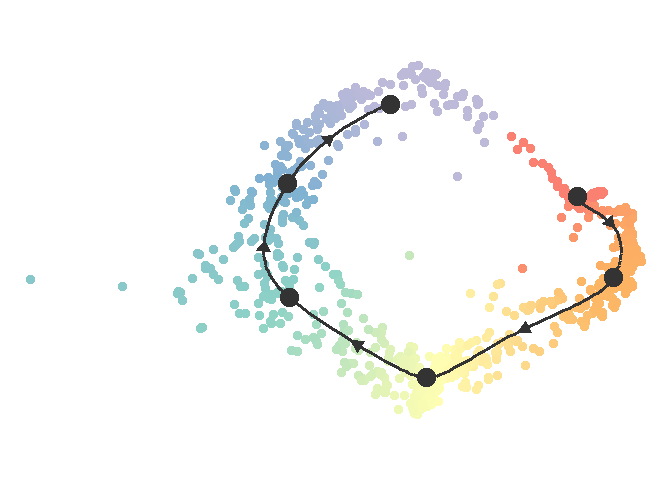In addition to the backbones already defined by `dyngen`, you can define your own custom backbone by using one of two ways.

### Manually

The first approach is to study the `?backbone` documentation. This will allow you to create any sort of backbone you like (disconnected, cyclic, converging, …), but also requires you to understand the backbone in detail and will typically involve experimenting with the different parameters a little bit.

This is an example of what data structures a backbone consists of.

``````backbone <- backbone_bifurcating_loop()

print(backbone\$module_info)``````
``````## # A tibble: 13 x 5
##    module_id basal burn  independence color
##    <chr>     <dbl> <lgl>        <dbl> <chr>
##  1 A1            1 TRUE             1 #FF9999
##  2 A2            0 TRUE             1 #FF4D4D
##  3 A3            1 TRUE             1 #FF0000
##  4 B1            0 FALSE            1 #CCFF99
##  5 B2            1 TRUE             1 #80FF00
##  6 C1            0 FALSE            1 #99FFFF
##  7 C2            0 FALSE            1 #4DFFFF
##  8 C3            0 FALSE            1 #00FFFF
##  9 D1            0 FALSE            1 #CC99FF
## 10 D2            0 FALSE            1 #B973FF
## 11 D3            1 TRUE             1 #A64DFF
## 12 D4            0 FALSE            1 #9326FF
## 13 D5            0 FALSE            1 #8000FF
``````
``print(backbone\$module_network)``
``````## # A tibble: 22 x 5
##    from  to    effect strength  hill
##    <chr> <chr>  <int>    <dbl> <dbl>
##  1 A1    A2         1       10     2
##  2 A2    A3        -1       10     2
##  3 A2    B1         1        1     2
##  4 B1    B2        -1       10     2
##  5 B1    C1         1        1     2
##  6 B1    D1         1        1     2
##  7 C1    C1         1       10     2
##  8 C1    D1        -1      100     2
##  9 C1    C2         1        1     2
## 10 C2    C3         1        1     2
## # … with 12 more rows
``````
``print(backbone\$expression_patterns)``
``````## # A tibble: 5 x 6
##   from  to    module_progression              start burn   time
##   <chr> <chr> <chr>                           <lgl> <lgl> <dbl>
## 1 sBurn sA    +A1,+A2,+A3,+B2,+D3             TRUE  TRUE     60
## 2 sA    sB    +B1                             FALSE FALSE    60
## 3 sB    sC    +C1,+C2|-A2,-B1,+C3|-C1,-D1,-D2 FALSE FALSE    80
## 4 sB    sD    +D1,+D2,+D4,+D5                 FALSE FALSE   120
## 5 sC    sA    +A1,+A2                         FALSE FALSE    60
``````

This allows you to simulate the following dataset.

``````out <-
initialise_model(
backbone = backbone,
num_tfs = 40,
num_targets = 0,
num_hks = 0,
verbose = FALSE,
num_cores =
) %>%
generate_dataset(make_plots = TRUE)``````
``print(out\$plot)``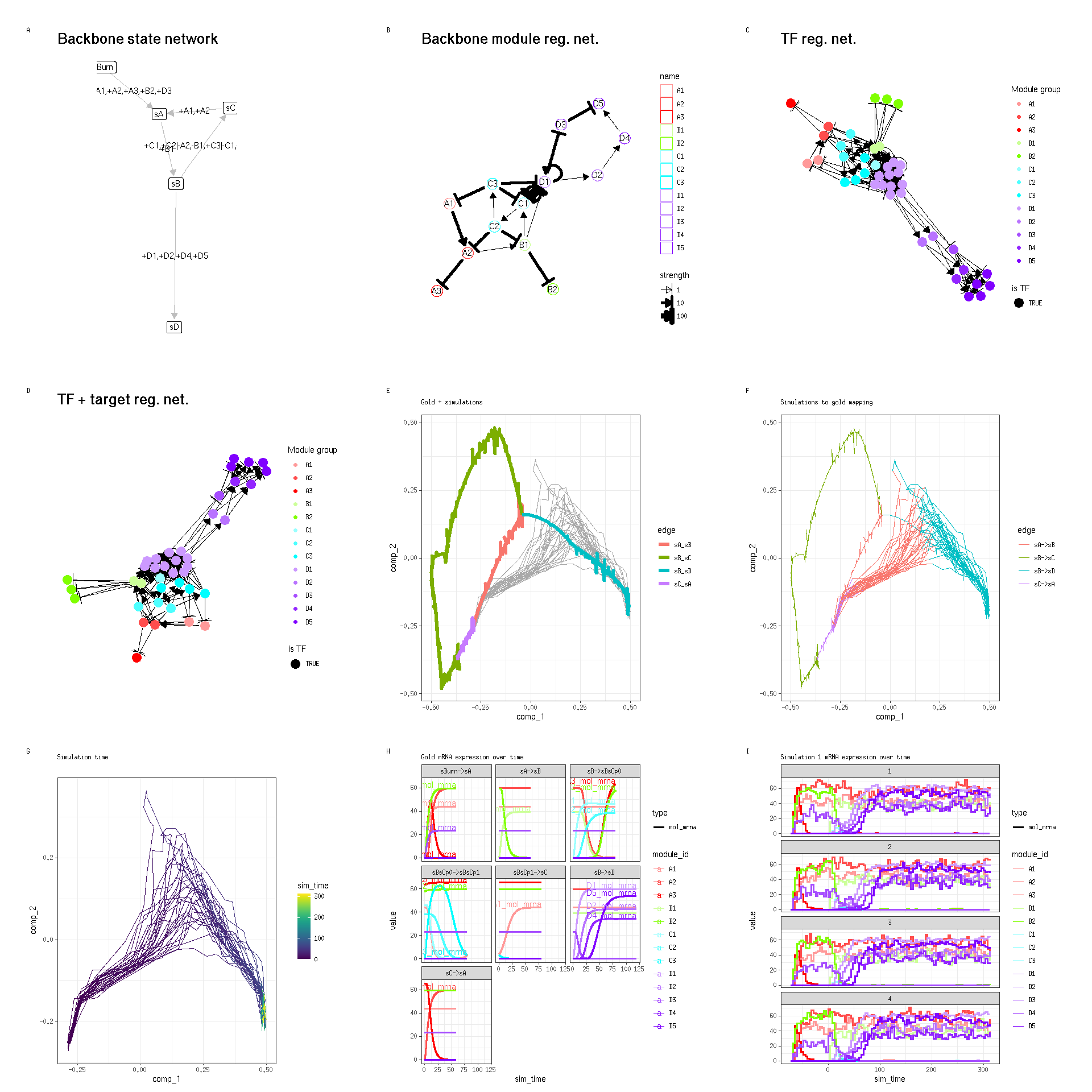### Backbone lego

Alternatively, you can use the `bblego` functions in order to create custom backbones using various components. Please note that the `bblego` functions currently only allow you to create tree-like backbones. See `?bblego` for more details.

Here is an example of a bifurcating trajectory.

``````backbone <- bblego(
bblego_start("A", type = "simple", num_modules = 2),
bblego_linear("A", "B", type = "flipflop", num_modules = 4),
bblego_branching("B", c("C", "D"), type = "simple"),
bblego_end("C", type = "doublerep2", num_modules = 4),
bblego_end("D", type = "doublerep1", num_modules = 7)
)

out <-
initialise_model(
backbone = backbone,
num_tfs = 40,
num_targets = 0,
num_hks = 0,
verbose = FALSE
) %>%
generate_dataset(make_plots = TRUE)``````
``print(out\$plot)``